# genrfeatures

Perform automated feature engineering for regression

## Syntax

``[Transformer,NewTbl] = genrfeatures(Tbl,ResponseVarName,q)``
``[Transformer,NewTbl] = genrfeatures(Tbl,Y,q)``
``[Transformer,NewTbl] = genrfeatures(Tbl,formula,q)``
``[Transformer,NewTbl] = genrfeatures(___,Name=Value)``

## Description

The `genrfeatures` function enables you to automate the feature engineering process in the context of a machine learning workflow. Before passing tabular training data to a regression model, you can create new features from the predictors in the data by using `genrfeatures`. Use the returned data to train the regression model.

`genrfeatures` allows you to generate features from variables with data types—such as `datetime`, `duration`, and various `int` types—that are not supported by most regression model training functions. The resulting features have data types that are supported by these training functions.

To better understand the generated features, use the `describe` function of the returned `FeatureTransformer` object. To apply the same training set feature transformations to a test set, use the `transform` function of the `FeatureTransformer` object.

example

````[Transformer,NewTbl] = genrfeatures(Tbl,ResponseVarName,q)` uses automated feature engineering to create `q` features from the predictors in `Tbl`. The software assumes that the `ResponseVarName` variable in `Tbl` is the response and does not create new features from this variable. `genrfeatures` returns a `FeatureTransformer` object (`Transformer`) and a new table (`NewTbl`) that contains the transformed features.By default, `genrfeatures` assumes that generated features are used to train an interpretable linear regression model. If you want to generate features to improve the accuracy of a bagged ensemble, specify `TargetLearner="bag"`.```
````[Transformer,NewTbl] = genrfeatures(Tbl,Y,q)` assumes that the vector `Y` is the response variable and creates new features from the variables in `Tbl`.```
````[Transformer,NewTbl] = genrfeatures(Tbl,formula,q)` uses the explanatory model `formula` to determine the response variable in `Tbl` and the subset of `Tbl` predictors from which to create new features.```

example

````[Transformer,NewTbl] = genrfeatures(___,Name=Value)` specifies options using one or more name-value arguments in addition to any of the input argument combinations in previous syntaxes. For example, you can change the expected learner type, the method for selecting new features, and the standardization method for transformed data.```

## Examples

collapse all

Use automated feature engineering to generate new features. Train a linear regression model using the generated features. Interpret the relationship between the generated features and the trained model.

Load the `patients` data set. Create a table from a subset of the variables. Display the first few rows of the table.

```load patients Tbl = table(Age,Diastolic,Gender,Height,SelfAssessedHealthStatus, ... Smoker,Weight,Systolic); head(Tbl)```
```ans=8×8 table Age Diastolic Gender Height SelfAssessedHealthStatus Smoker Weight Systolic ___ _________ __________ ______ ________________________ ______ ______ ________ 38 93 {'Male' } 71 {'Excellent'} true 176 124 43 77 {'Male' } 69 {'Fair' } false 163 109 38 83 {'Female'} 64 {'Good' } false 131 125 40 75 {'Female'} 67 {'Fair' } false 133 117 49 80 {'Female'} 64 {'Good' } false 119 122 46 70 {'Female'} 68 {'Good' } false 142 121 33 88 {'Female'} 64 {'Good' } true 142 130 40 82 {'Male' } 68 {'Good' } false 180 115 ```

Generate 10 new features from the variables in `Tbl`. Specify the `Systolic` variable as the response. By default, `genrfeatures` assumes that the new features will be used to train a linear regression model.

```rng("default") % For reproducibility [T,NewTbl] = genrfeatures(Tbl,"Systolic",10)```
```T = FeatureTransformer with properties: Type: 'regression' TargetLearner: 'linear' NumEngineeredFeatures: 10 NumOriginalFeatures: 0 TotalNumFeatures: 10 ```
```NewTbl=100×11 table zsc(d(Smoker)) q8(Age) eb8(Age) zsc(sin(Height)) zsc(kmd8) q6(Height) eb8(Diastolic) q8(Diastolic) zsc(fenc(c(SelfAssessedHealthStatus))) q10(Weight) Systolic ______________ _______ ________ ________________ _________ __________ ______________ _____________ ______________________________________ ___________ ________ 1.3863 4 5 1.1483 -0.56842 6 8 8 0.27312 7 124 -0.71414 6 6 -0.3877 -2.0772 5 2 2 -1.4682 6 109 -0.71414 4 5 1.1036 -0.21519 2 4 5 0.82302 3 125 -0.71414 5 6 -1.4552 -0.32389 4 2 2 -1.4682 4 117 -0.71414 8 8 1.1036 1.2302 2 3 4 0.82302 1 122 -0.71414 7 7 -1.5163 -0.88497 4 1 1 0.82302 5 121 1.3863 3 3 1.1036 -1.1434 2 6 6 0.82302 5 130 -0.71414 5 6 -1.5163 -0.3907 4 4 5 0.82302 8 115 -0.71414 1 2 -1.5163 0.4278 4 3 3 0.27312 9 115 -0.71414 2 3 -0.26055 -0.092621 3 5 6 0.27312 3 118 -0.71414 7 7 -1.5163 0.16737 4 2 2 0.27312 2 114 -0.71414 6 6 -0.26055 -0.32104 3 1 1 -1.8348 5 115 -0.71414 1 1 1.1483 -0.051074 6 1 1 -1.8348 7 127 1.3863 5 5 0.14351 2.3695 6 8 8 0.27312 10 130 -0.71414 3 4 0.96929 0.092962 2 3 4 0.82302 3 114 1.3863 8 8 1.1483 -0.049336 6 7 8 0.82302 8 130 ⋮ ```

`T` is a `FeatureTransformer` object that can be used to transform new data, and `newTbl` contains the new features generated from the `Tbl` data.

To better understand the generated features, use the `describe` object function of the `FeatureTransformer` object. For example, inspect the first two generated features.

`describe(T,1:2)`
``` Type IsOriginal InputVariables Transformations ___________ __________ ______________ ___________________________________________________________ zsc(d(Smoker)) Numeric false Smoker Variable of type double converted from an integer data type Standardization with z-score (mean = 0.34, std = 0.4761) q8(Age) Categorical false Age Equiprobable binning (number of bins = 8) ```

The first feature in `newTbl` is a numeric variable, created by first converting the values of the `Smoker` variable to a numeric variable of type `double` and then transforming the results to z-scores. The second feature in `newTbl` is a categorical variable, created by binning the values of the `Age` variable into 8 equiprobable bins.

Use the generated features to fit a linear regression model without any regularization.

`Mdl = fitrlinear(NewTbl,"Systolic",Lambda=0);`

Plot the coefficients of the predictors used to train `Mdl`. Note that `fitrlinear` expands categorical predictors before fitting a model.

```p = length(Mdl.Beta); [sortedCoefs,expandedIndex] = sort(Mdl.Beta,ComparisonMethod="abs"); sortedExpandedPreds = Mdl.ExpandedPredictorNames(expandedIndex); bar(sortedCoefs,Horizontal="on") yticks(1:2:p) yticklabels(sortedExpandedPreds(1:2:end)) xlabel("Coefficient") ylabel("Expanded Predictors") title("Coefficients for Expanded Predictors")```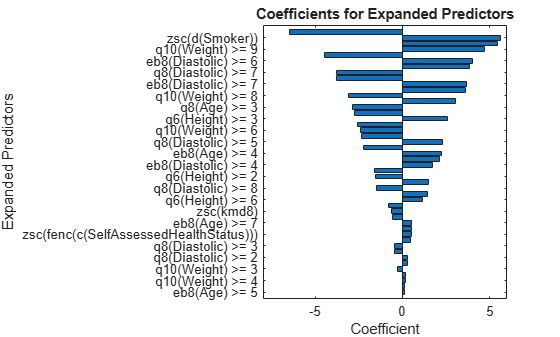Identify the predictors whose coefficients have larger absolute values.

```bigCoefs = abs(sortedCoefs) >= 4; flip(sortedExpandedPreds(bigCoefs))```
```ans = 1x6 cell Columns 1 through 3 {'eb8(Diastolic)...'} {'zsc(d(Smoker))'} {'q8(Age) >= 2'} Columns 4 through 6 {'q10(Weight) >= 9'} {'q6(Height) >= 5'} {'eb8(Diastolic)...'} ```

You can use partial dependence plots to analyze the categorical features whose levels have large coefficients in terms of absolute value. For example, inspect the partial dependence plot for the `eb8(Diastolic)` variable, whose levels `eb8(Diastolic) >= 5` and `eb8(Diastolic) >= 6` have coefficients with large absolute values. These two levels correspond to noticeable changes in the predicted `Systolic` values.

`plotPartialDependence(Mdl,"eb8(Diastolic)",NewTbl);`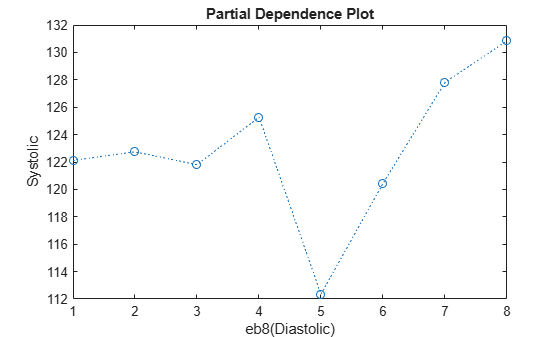Generate new features to improve the predictive performance of an interpretable linear regression model. Compare the test set performance of a linear model trained on the original data to the test set performance of a linear model trained on the transformed features.

Load the `carbig` data set, which contains measurements of cars made in the 1970s and early 1980s.

`load carbig`

Convert the `Origin` variable to a categorical variable. Then create a table containing the predictor variables `Acceleration`, `Displacement`, and so on, as well as the response variable `MPG`. Each row contains the measurements for a single car. Remove rows that have missing values.

```Origin = categorical(cellstr(Origin)); cars = table(Acceleration,Displacement,Horsepower, ... Model_Year,Origin,Weight,MPG); Tbl = rmmissing(cars);```

Partition the data into training and test sets. Use approximately 70% of the observations as training data, and 30% of the observations as test data. Partition the data using `cvpartition`.

```rng("default") % For reproducibility of the partition c = cvpartition(size(Tbl,1),Holdout=0.3); trainIdx = training(c); trainTbl = Tbl(trainIdx,:); testIdx = test(c); testTbl = Tbl(testIdx,:);```

Use the training data to generate 45 new features. Inspect the returned `FeatureTransformer` object.

```[T,newTrainTbl] = genrfeatures(trainTbl,"MPG",45); T```
```T = FeatureTransformer with properties: Type: 'regression' TargetLearner: 'linear' NumEngineeredFeatures: 43 NumOriginalFeatures: 2 TotalNumFeatures: 45 ```

Note that `T.NumOriginalFeatures` is `2`, which means the function keeps two of the original predictors.

Apply the transformations stored in the object `T` to the test data.

`newTestTbl = transform(T,testTbl);`

Compare the test set performances of a linear model trained on the original features and a linear model trained on the new features.

Train a linear regression model using the original training set `trainTbl`, and compute the mean squared error (MSE) of the model on the original test set `testTbl`. Then, train a linear regression model using the transformed training set `newTrainTbl`, and compute the MSE of the model on the transformed test set `newTestTbl`.

```originalMdl = fitrlinear(trainTbl,"MPG"); originalTestMSE = loss(originalMdl,testTbl,"MPG")```
```originalTestMSE = 65.9916 ```
```newMdl = fitrlinear(newTrainTbl,"MPG"); newTestMSE = loss(newMdl,newTestTbl,"MPG")```
```newTestMSE = 12.3329 ```

`newTestMSE` is less than `originalTestMSE`, which suggests that the linear model trained on the transformed data performs better than the linear model trained on the original data.

Compare the predicted test set response values to the true response values for both models. Plot the predicted miles per gallon (MPG) along the vertical axis and the true MPG along the horizontal axis. Points on the reference line indicate correct predictions. A good model produces predictions that are scattered near the line.

```predictedTestY = predict(originalMdl,testTbl); newPredictedTestY = predict(newMdl,newTestTbl); plot(testTbl.MPG,predictedTestY,".") hold on plot(testTbl.MPG,newPredictedTestY,".") hold on plot(testTbl.MPG,testTbl.MPG) hold off xlabel("True Miles Per Gallon (MPG)") ylabel("Predicted Miles Per Gallon (MPG)") legend(["Original Model Results","New Model Results","Reference Line"])```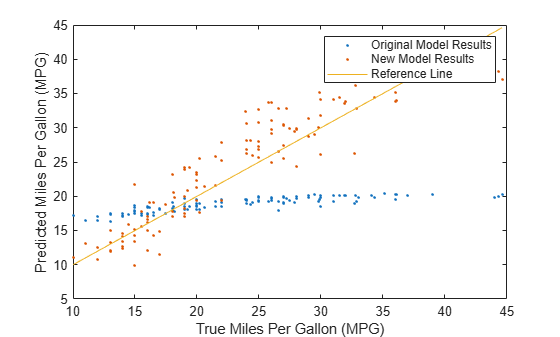Use `genrfeatures` to engineer new features before training a bagged ensemble regression model. Before making predictions on new data, apply the same feature transformations to the new data set. Compare the test set performance of the ensemble that uses the engineered features to the test set performance of the ensemble that uses the original features.

Read power outage data into the workspace as a table. Remove observations with missing values, and display the first few rows of the table.

```outages = readtable("outages.csv"); Tbl = rmmissing(outages); head(Tbl)```
```ans=8×6 table Region OutageTime Loss Customers RestorationTime Cause _____________ ________________ ______ __________ ________________ ___________________ {'SouthWest'} 2002-02-01 12:18 458.98 1.8202e+06 2002-02-07 16:50 {'winter storm' } {'SouthEast'} 2003-02-07 21:15 289.4 1.4294e+05 2003-02-17 08:14 {'winter storm' } {'West' } 2004-04-06 05:44 434.81 3.4037e+05 2004-04-06 06:10 {'equipment fault'} {'MidWest' } 2002-03-16 06:18 186.44 2.1275e+05 2002-03-18 23:23 {'severe storm' } {'West' } 2003-06-18 02:49 0 0 2003-06-18 10:54 {'attack' } {'NorthEast'} 2003-07-16 16:23 239.93 49434 2003-07-17 01:12 {'fire' } {'MidWest' } 2004-09-27 11:09 286.72 66104 2004-09-27 16:37 {'equipment fault'} {'SouthEast'} 2004-09-05 17:48 73.387 36073 2004-09-05 20:46 {'equipment fault'} ```

Some of the variables, such as `OutageTime` and `RestorationTime`, have data types that are not supported by regression model training functions like `fitrensemble`.

Partition the data into training and test sets. Use approximately 70% of the observations as training data, and 30% of the observations as test data. Partition the data using `cvpartition`.

```rng("default") % For reproducibility of the partition c = cvpartition(size(Tbl,1),Holdout=0.30); TrainTbl = Tbl(training(c),:); TestTbl = Tbl(test(c),:);```

Use the training data to generate 30 new features to fit a bagged ensemble. By default, the 30 features include original features that can be used as predictors by a bagged ensemble.

```[Transformer,NewTrainTbl] = genrfeatures(TrainTbl,"Loss",30, ... TargetLearner="bag"); Transformer```
```Transformer = FeatureTransformer with properties: Type: 'regression' TargetLearner: 'bag' NumEngineeredFeatures: 27 NumOriginalFeatures: 3 TotalNumFeatures: 30 ```

Create `NewTestTbl` by applying the transformations stored in the object `Transformer` to the test data.

`NewTestTbl = transform(Transformer,TestTbl);`

Train a bagged ensemble using the original training set `TrainTbl`, and compute the mean squared error (MSE) of the model on the original test set `TestTbl`. Specify only the three predictor variables that can be used by `fitrensemble` (`Region`, `Customers`, and `Cause`), and omit the two `datetime` predictor variables (`OutageTime` and `RestorationTime`). Then, train a bagged ensemble using the transformed training set `NewTrainTbl`, and compute the MSE of the model on the transformed test set `NewTestTbl`.

```originalMdl = fitrensemble(TrainTbl,"Loss ~ Region + Customers + Cause", ... Method="bag"); originalTestMSE = loss(originalMdl,TestTbl)```
```originalTestMSE = 1.8999e+06 ```
```newMdl = fitrensemble(NewTrainTbl,"Loss",Method="bag"); newTestMSE = loss(newMdl,NewTestTbl)```
```newTestMSE = 1.8617e+06 ```

`newTestMSE` is less than `originalTestMSE`, which suggests that the bagged ensemble trained on the transformed data performs slightly better than the bagged ensemble trained on the original data.

Compare the predicted test set response values to the true response values for both models. Plot the log of the predicted response along the vertical axis and the log of the true response (`Loss`) along the horizontal axis. Points on the reference line indicate correct predictions. A good model produces predictions that are scattered near the line.

```predictedTestY = predict(originalMdl,TestTbl); newPredictedTestY = predict(newMdl,NewTestTbl); plot(log(TestTbl.Loss),log(predictedTestY),".") hold on plot(log(TestTbl.Loss),log(newPredictedTestY),".") hold on plot(log(TestTbl.Loss),log(TestTbl.Loss)) hold off xlabel("log(True Response)") ylabel("log(Predicted Response)") legend(["Original Model Results","New Model Results","Reference Line"], ... Location="southeast") xlim([-1 10]) ylim([-1 10])```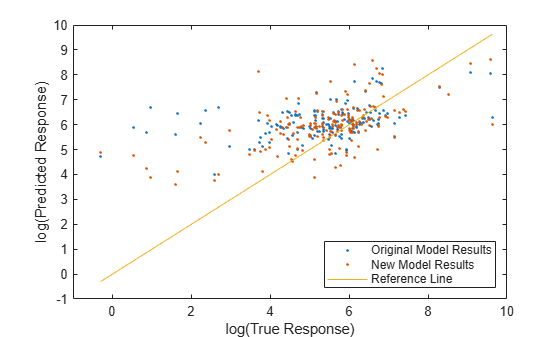Engineer and inspect new features before training a support vector machine (SVM) regression model with a Gaussian kernel. Then, assess the test set performance of the model.

Load the `carbig` data set, which contains measurements of cars made in the 1970s and early 1980s.

`load carbig`

Create a table containing the numeric predictor variables `Acceleration`, `Displacement`, and so on, as well as the response variable `MPG`. Each row contains the measurements for a single car. Remove rows that have missing values.

```cars = table(Acceleration,Displacement,Horsepower, ... Model_Year,Weight,MPG); Tbl = rmmissing(cars); head(Tbl)```
```ans=8×6 table Acceleration Displacement Horsepower Model_Year Weight MPG ____________ ____________ __________ __________ ______ ___ 12 307 130 70 3504 18 11.5 350 165 70 3693 15 11 318 150 70 3436 18 12 304 150 70 3433 16 10.5 302 140 70 3449 17 10 429 198 70 4341 15 9 454 220 70 4354 14 8.5 440 215 70 4312 14 ```

Partition the data into training and test sets. Use approximately 75% of the observations as training data, and 25% of the observations as test data. Partition the data using `cvpartition`.

```rng("default") % For reproducibility of the partition n = length(Tbl.MPG); c = cvpartition(n,Holdout=0.25); trainTbl = Tbl(training(c),:); testTbl = Tbl(test(c),:);```

Use the training data to generate 25 features to fit an SVM regression model with a Gaussian kernel. By default, the 25 features include original features that can be used as predictors by an SVM regression model. Additionally, `genrfeatures` uses neighborhood component analysis (NCA) to reduce the set of engineered features to the most important predictors. You can use the NCA feature selection method only when the target learner is `"gaussian-svm"` and all the predictor features are numeric.

```[Transformer,newTrainTbl] = genrfeatures(trainTbl,"MPG",25, ... TargetLearner="gaussian-svm")```
```Transformer = FeatureTransformer with properties: Type: 'regression' TargetLearner: 'gaussian-svm' NumEngineeredFeatures: 20 NumOriginalFeatures: 5 TotalNumFeatures: 25 ```
```newTrainTbl=294×26 table zsc(Acceleration) zsc(Displacement) zsc(Horsepower) zsc(Model_Year) zsc(Weight) zsc(Acceleration.*Horsepower) zsc(Acceleration.*Weight) zsc(Horsepower.*Weight) zsc(Model_Year.*Weight) zsc(Acceleration+Model_Year) zsc(Acceleration-Model_Year) zsc(Displacement./Horsepower) zsc(Displacement./Weight) zsc(Model_Year./Weight) zsc(kmc4) zsc(kmc5) zsc(sin(Acceleration)) zsc(sin(Weight)) zsc(cos(Displacement)) zsc(cos(Horsepower)) zsc(cos(Model_Year)) zsc(cos(Weight)) zsc(log(Displacement)) zsc(log(Horsepower)) zsc(log(Model_Year)) MPG _________________ _________________ _______________ _______________ ___________ _____________________________ _________________________ _______________________ _______________________ ____________________________ ____________________________ _____________________________ _________________________ _______________________ _________ _________ ______________________ ________________ ______________________ ____________________ ____________________ ________________ ______________________ ____________________ ____________________ ___ -1.2878 1.0999 0.67715 -1.6278 0.6473 0.046384 -0.25776 0.54695 0.35655 -1.8529 0.58974 1.1707 1.4711 -0.98922 -0.27892 0.58093 -0.84061 -1.2446 1.0834 -0.52084 0.88679 -0.63765 1.1489 0.83573 -1.6691 18 -1.4652 1.5106 1.5694 -1.6278 0.87016 0.88366 -0.22352 1.2406 0.57391 -1.9492 0.46488 0.68641 1.8668 -1.1166 -0.72987 1.0942 -1.3283 -1.379 -0.27316 -0.099711 0.88679 0.063193 1.3943 1.5178 -1.6691 15 -1.6425 1.2049 1.187 -1.6278 0.56711 0.26966 -0.60316 0.81696 0.27835 -2.0455 0.34001 0.68397 1.7439 -0.93998 -0.27892 0.58093 -1.5076 -1.0814 -0.98059 0.97158 0.88679 0.86743 1.2148 1.2451 -1.6691 18 -1.8198 1.0521 0.93209 -1.6278 0.58244 -0.17689 -0.73163 0.67023 0.2933 -2.1417 0.21515 0.75883 1.4682 -0.94955 -0.27892 0.58093 -1.3344 -0.61728 1.4918 -0.28369 0.88679 1.2572 1.1182 1.0477 -1.6691 17 -1.9971 2.2653 2.4107 -1.6278 1.6343 1.0883 -0.14713 2.3686 1.3191 -2.238 0.090291 0.77802 2.0908 -1.469 -1.7817 1.6098 -0.85133 -0.86513 -0.10886 -1.4017 0.88679 1.0923 1.7752 2.0394 -1.6691 15 -2.3517 2.5041 2.9716 -1.6278 1.6496 1.0883 -0.49025 2.8122 1.3341 -2.4305 -0.15943 0.57038 2.3918 -1.475 -1.7817 1.6098 0.52473 -0.329 0.084822 1.3869 0.88679 1.368 1.8812 2.3408 -1.6691 14 -2.529 2.3704 2.8441 -1.6278 1.6001 0.71 -0.69609 2.6733 1.2858 -2.5268 -0.28429 0.53587 2.2685 -1.4555 -1.7817 1.6098 1.0808 1.3872 1.5888 0.26987 0.88679 -0.2533 1.8226 2.275 -1.6691 14 -2.529 1.8927 2.2068 -1.6278 1.0553 0.18283 -1.0151 1.7914 0.75446 -2.5268 -0.28429 0.54821 2.2275 -1.2128 -0.72987 1.0942 1.0808 -1.381 1.4703 0.085898 0.88679 -0.050724 1.5968 1.9214 -1.6691 15 -1.9971 1.8259 1.6969 -1.6278 0.71687 0.3937 -0.77911 1.2242 0.4244 -2.238 0.090291 0.95189 2.5699 -1.0304 -0.27892 0.58093 -0.85133 0.59822 1.5569 1.3056 0.88679 1.279 1.5629 1.6032 -1.6691 15 -2.7063 1.4151 1.442 -1.6278 0.77111 -0.64825 -1.3281 1.0967 0.4773 -2.623 -0.40916 0.69405 1.8356 -1.0616 -0.27892 0.58093 1.3555 0.89932 1.2589 -1.3721 0.88679 -1.1233 1.34 1.4297 -1.6691 14 -1.9971 2.5137 3.0991 -1.6278 0.1544 1.7582 -1.1666 1.6239 -0.12417 -2.238 0.090291 0.48692 4.7777 -0.65221 0.35951 0.08908 -0.85133 1.1507 -1.1229 0.5071 0.88679 0.80592 1.8853 2.4051 -1.6691 14 -0.22399 -0.75341 -0.21514 -1.6278 -0.68753 -0.28853 -0.78317 -0.49096 -0.9453 -1.2754 1.3389 -1.1913 -0.73834 0.19818 0.38679 -0.64629 0.8675 -0.12343 1.6048 1.0148 0.88679 -1.4439 -0.72174 -0.0616 -1.6691 24 -0.046679 0.058585 -0.21514 -1.6278 -0.14393 -0.17069 -0.10639 -0.29348 -0.41513 -1.1791 1.4638 0.61185 0.49152 -0.39991 0.8353 -0.33885 0.22876 -0.90303 -1.3204 1.0148 0.88679 1.0596 0.32804 -0.0616 -1.6691 22 0.13063 0.077691 -0.47008 -1.6278 -0.43401 -0.44978 -0.31105 -0.51551 -0.69804 -1.0829 1.5886 1.1534 0.90157 -0.10728 0.8353 -0.33885 -0.48273 -1.3741 0.85875 -1.3843 0.88679 -0.16461 0.34685 -0.3798 -1.6691 21 1.7264 -0.90626 -1.4643 -1.6278 -1.3207 -1.4843 -0.61766 -1.1264 -1.5629 -0.21655 2.7124 0.66119 -0.44972 1.2738 -0.97737 -1.3862 1.3662 0.43728 -1.2152 -0.61163 0.88679 1.3434 -1.0075 -2.1364 -1.6691 26 0.66256 -0.83939 -0.21514 -1.6278 -0.68399 0.30067 -0.2972 -0.48967 -0.94185 -0.7941 1.9632 -1.3822 -0.95111 0.19354 0.38679 -0.64629 -1.4725 -0.049071 -1.2471 1.0148 0.88679 1.4102 -0.87708 -0.0616 -1.6691 25 ⋮ ```

By default, `genrfeatures` standardizes the original features before including them in `newTrainTbl`.

Inspect the first three engineered features. Note that the engineered features are stored after the five original features in the `Transformer` object. Visualize the engineered features by using a matrix of scatter plots and histograms.

```featIndex = 6:8; describe(Transformer,featIndex)```
``` Type IsOriginal InputVariables Transformations _______ __________ ________________________ ____________________________________________________________________ zsc(Acceleration.*Horsepower) Numeric false Acceleration, Horsepower Acceleration .* Horsepower Standardization with z-score (mean = 1541.3031, std = 403.0917) zsc(Acceleration.*Weight) Numeric false Acceleration, Weight Acceleration .* Weight Standardization with z-score (mean = 45221.1823, std = 12310.4734) zsc(Horsepower.*Weight) Numeric false Horsepower, Weight Horsepower .* Weight Standardization with z-score (mean = 334221.0068, std = 221771.6874) ```
`plotmatrix(newTrainTbl{:,featIndex})`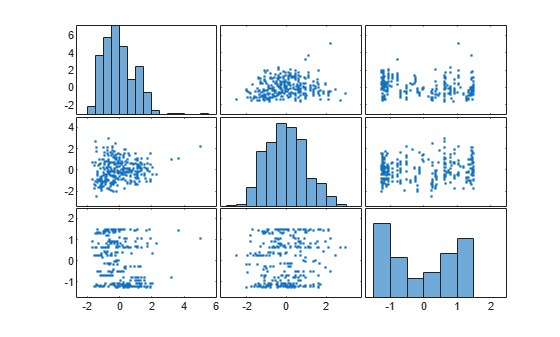The plots can help you better understand the engineered features. For example:

• The top-left plot is a histogram of the `zsc(Acceleration.*Horsepower)` feature. This feature consists of the standardized element-wise product of the original `Acceleration` and `Horsepower` features. The histogram shows that `zsc(Acceleration.*Horsepower)` has a few outlying values greater than 3.

• The bottom-left plot is a scatter plot that compares the `zsc(Acceleration.*Horsepower)` values (along the x-axis) to the `zsc(Horsepower.*Weight)` values (along the y-axis). The scatter plot shows that the `zsc(Horsepower.*Weight)` values tend to increase as the `zsc(Acceleration.*Horsepower)` values increase. Note that this plot contains the same information as the top-right plot, but with the axes flipped.

Create `newTestTbl` by applying the transformations stored in the object `Transformer` to the test data.

`newTestTbl = transform(Transformer,testTbl);`

Train an SVM regression model with a Gaussian kernel using the transformed training set `newTrainTbl`. Let the `fitrsvm` function find an appropriate scale value for the kernel function. Compute the mean squared error (MSE) of the model on the transformed test set `newTestTbl`.

```Mdl = fitrsvm(newTrainTbl,"MPG",KernelFunction="gaussian", ... KernelScale="auto"); testMSE = loss(Mdl,newTestTbl,"MPG")```
```testMSE = 9.4666 ```

Compare the predicted test set response values to the true response values. Plot the predicted miles per gallon (MPG) along the vertical axis and the true MPG along the horizontal axis. Points on the reference line indicate correct predictions. A good model produces predictions that are scattered near the line.

```predictedTestY = predict(Mdl,newTestTbl); plot(newTestTbl.MPG,predictedTestY,".") hold on plot(newTestTbl.MPG,newTestTbl.MPG) hold off xlabel("True Miles Per Gallon (MPG)") ylabel("Predicted Miles Per Gallon (MPG)")```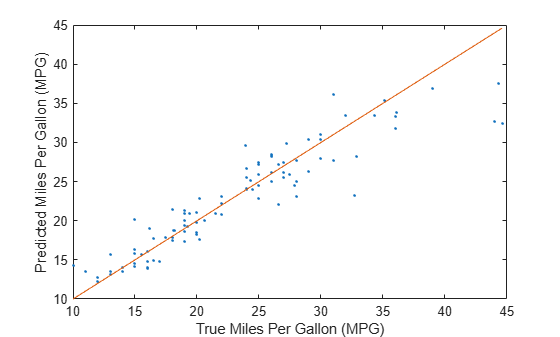The SVM model seems to predict MPG values well.

Generate features to train a linear regression model. Compute the cross-validation mean squared error (MSE) of the model by using the `crossval` function.

Load the `patients` data set, and create a table containing the predictor data.

```load patients Tbl = table(Age,Diastolic,Gender,Height,SelfAssessedHealthStatus, ... Smoker,Weight);```

Create a random partition for 5-fold cross-validation.

```rng("default") % For reproducibility of the partition cvp = cvpartition(size(Tbl,1),KFold=5);```

Compute the cross-validation MSE for a linear regression model trained on the original features in `Tbl` and the `Systolic` response variable.

```CVMdl = fitrlinear(Tbl,Systolic,CVPartition=cvp); cvloss = kfoldLoss(CVMdl)```
```cvloss = 45.2594 ```

Create the custom function `myloss` (shown at the end of this example). This function generates 20 features from the training data, and then applies the same training set transformations to the test data. The function then fits a linear regression model to the training data and computes the test set MSE.

Note: If you use the live script file for this example, the `myloss` function is already included at the end of the file. Otherwise, you need to create this function at the end of your .m file or add it as a file on the MATLAB® path.

Compute the cross-validation MSE for a linear model trained on features generated from the predictors in `Tbl`.

`newcvloss = mean(crossval(@myloss,Tbl,Systolic,Partition=cvp))`
```newcvloss = 26.9429 ```
```function testloss = myloss(TrainTbl,trainY,TestTbl,testY) [Transformer,NewTrainTbl] = genrfeatures(TrainTbl,trainY,20); NewTestTbl = transform(Transformer,TestTbl); Mdl = fitrlinear(NewTrainTbl,trainY); testloss = loss(Mdl,NewTestTbl,testY); end```

## Input Arguments

collapse all

Original features, specified as a table. Each row of `Tbl` corresponds to one observation, and each column corresponds to one predictor variable. Optionally, `Tbl` can contain one additional column for the response variable. Multicolumn variables and cell arrays other than cell arrays of character vectors are not allowed, but `datetime`, `duration`, and various `int` predictor variables are allowed.

• If `Tbl` contains the response variable, and you want to create new features from any of the remaining variables in `Tbl`, then specify the response variable by using `ResponseVarName`.

• If `Tbl` contains the response variable, and you want to create new features from only a subset of the remaining variables in `Tbl`, then specify a formula by using `formula`.

• If `Tbl` does not contain the response variable, then specify a response variable by using `Y`. The length of the response variable and the number of rows in `Tbl` must be equal.

Data Types: `table`

Response variable name, specified as the name of a variable in `Tbl`.

You must specify `ResponseVarName` as a character vector or string scalar. For example, if the response variable `Y` is stored as `Tbl.Y`, then specify it as `'Y'`. Otherwise, the software treats all columns of `Tbl` as predictors, and might create new features from `Y`.

Data Types: `char` | `string`

Number of features, specified as a positive integer scalar. For example, you can set `q` to approximately `1.5*size(Tbl,2)`, which is about 1.5 times the number of original features.

Data Types: `single` | `double`

Response variable, specified as a numeric column vector. `Y` and `Tbl` must have the same number of rows.

Data Types: `single` | `double`

Explanatory model of the response variable and a subset of the predictor variables, specified as a character vector or string scalar in the form `"Y~X1+X2+X3"`. In this form, `Y` represents the response variable, and `X1`, `X2`, and `X3` represent the predictor variables.

To create new features from only a subset of the predictor variables in `Tbl`, use a formula. If you specify a formula, then the software does not create new features from any variables in `Tbl` that do not appear in `formula`.

The variable names in the formula must be both variable names in `Tbl` (`Tbl.Properties.VariableNames`) and valid MATLAB® identifiers. You can verify the variable names in `Tbl` by using the `isvarname` function. If the variable names are not valid, then you can convert them by using the `matlab.lang.makeValidName` function.

Data Types: `char` | `string`

### Name-Value Arguments

Specify optional pairs of arguments as `Name1=Value1,...,NameN=ValueN`, where `Name` is the argument name and `Value` is the corresponding value. Name-value arguments must appear after other arguments, but the order of the pairs does not matter.

Example: `genrfeatures(Tbl,"Response",10,TargetLearner="bag",FeatureSelection="oob")` specifies that the expected learner type is a bagged ensemble regression model and the method for selecting features is an out-of-bag, predictor importance technique.

Expected learner type, specified as `"linear"`, `"bag"`, or `"gaussian-svm"`. The software creates and selects new features assuming they will be used to train this type of model.

ValueExpected Model
`"linear"``RegressionLinear` — You can create a model by using the `fitrlinear` function.
`"bag"``RegressionBaggedEnsemble` — You can create a model by using the `fitrensemble` function and specifying `Method="Bag"`.
`"gaussian-svm"``RegressionSVM` (with a Gaussian kernel) — You can create a model by using the `fitrsvm` function and specifying `KernelFunction="gaussian"`. To create a model with good predictive performance, specify `KernelScale="auto"`.

Example: `TargetLearner="bag"`

Method for including the original features in `Tbl` in the new table `NewTbl`, specified as one of the values in this table.

ValueDescription
`"auto"`

This value is equivalent to:

• `"select"` when `TargetLearner` is `"linear"`

• `"include"` when `TargetLearner` is `"bag"` or `"gaussian-svm"`

`"include"`

The software includes original features that can be used as predictors by the target learner, and excludes features that are:

• Unsupported, such as `datetime` and `duration` variables

• Constant-valued, including variables with all missing values

• Numeric with `NaN` or `Inf` values (when the `TargetLearner` is `"linear"` or `"gaussian-svm"`)

• Categorical with all unique values

• Categorical with more categories than the `CategoricalEncodingLimit` value

`"select"`The software includes original features that are supported by the target learner and considered to be important by the specified feature selection method (`FeatureSelectionMethod`).
`"omit"`The software omits the original features.

Note that the software applies the standardization method specified by the `TransformedDataStandardization` name-value argument to original features included in `NewTbl`.

Example: `IncludeInputVariables="include"`

Method for selecting new features, specified as one of the values in this table. The software generates many features using various transformations and uses this method to select the important features to include in `NewTbl`.

ValueDescription
`"auto"`

This value is equivalent to:

• `"lasso"` when `TargetLearner` is `"linear"`

• `"oob"` when `TargetLearner` is `"bag"`

• `"nca"` when `TargetLearner` is `"gaussian-svm"`

`"lasso"`

Lasso regularization — Available when `TargetLearner` is `"linear"`

To perform feature selection, the software uses `fitrlinear` with `Regularization` specified as `"lasso"`. The `fitrlinear` function uses a vector of regularization strengths (`Lambda`) to find a linear fit that has the requested number of features with nonzero coefficients (`Beta`). The software includes these important features in `NewTbl`.

`"oob"`

Out-of-bag, predictor importance estimates by permutation — Available when `TargetLearner` is `"bag"`

To perform feature selection, the software fits a bagged ensemble of trees and uses the `oobPermutedPredictorImportance` function to rank the features in the ensemble. The software includes the requested number of top-ranked features in `NewTbl`.

`"nca"`

Neighborhood component analysis (NCA) — Available when `TargetLearner` is `"gaussian-svm"` and the predictor features in `Tbl` are numeric only

Tip

If `TargetLearner` is `"gaussian-svm"` and `Tbl` includes categorical predictors, specify `FeatureSelectionMethod` as `"mrmr"`.

To perform feature selection, the software uses `fsrnca` to fit a `FeatureSelectionNCARegression` object, and then sorts the features by their average weights (`FeatureWeights`). Greater weight indicates greater feature importance. The software includes the requested number of important features in `NewTbl`.

`"mrmr"`

Minimum redundancy maximum relevance (MRMR) — Available when `TargetLearner` is `"linear"`, `"bag"`, or `"gaussian-svm"`

To perform feature selection, the software uses `fsrmrmr` to rank the features, and then includes the requested number of top-ranked features in `NewTbl`.

For more information on different feature selection methods, see Introduction to Feature Selection.

Example: `FeatureSelection="mrmr"`

Standardization method for the transformed data, specified as one of the values in this table. The software applies this standardization method to both engineered features and original features.

ValueDescription
`"auto"`

This value is equivalent to:

• `"zscore"` when `TargetLearner` is `"linear"` or `"gaussian-svm"`

• `"none"` when `TargetLearner` is `"bag"`

`"zscore"`Center and scale to have mean 0 and standard deviation 1
`"none"`Use raw data
`"mad"`Center and scale to have median 0 and median absolute deviation 1
`"range"`Scale range of data to [0,1]

Example: `TransformedDataStandardization="range"`

Maximum number of categories allowed in a categorical predictor, specified as a nonnegative integer scalar. If a categorical predictor has more than the specified number of categories, then `genrfeatures` does not create new features from the predictor and excludes the predictor from the new table `NewTbl`. The default value is `50` when `TargetLearner` is `"linear"` or `"gaussian-svm"`, and `Inf` when `TargetLearner` is `"bag"`.

Example: `CategoricalEncodingLimit=20`

Data Types: `single` | `double`

## Output Arguments

collapse all

Engineered feature transformer, returned as a `FeatureTransformer` object. To better understand the engineered features, use the `describe` object function of `Transformer`. To apply the same feature transformations on a new data set, use the `transform` object function of `Transformer`.

Generated features, returned as a table. Each row corresponds to an observation, and each column corresponds to a generated feature. If the response variable is included in `Tbl`, then `NewTbl` also includes the response variable. Use this table to train a regression model of type `TargetLearner`.

`NewTbl` contains generated features in the following order: original features, engineered features as ranked by the feature selection method, and the response variable.

## Tips

• By default, when `TargetLearner` is `"linear"` or `"gaussian-svm"`, the software generates new features from numeric predictors by using z-scores (see `TransformedDataStandardization`). You can change the type of standardization for the transformed features. However, using some method of standardization, thereby avoiding the `"none"` specification, is strongly recommended. Fitting linear and SVM models works best with standardized data.

• When you generate features to create an SVM model with good predictive performance, specify `KernelScale` as `"auto"` in the call to `fitrsvm`. This specification allows the software to find an appropriate scale value for the SVM kernel function.

## Version History

Introduced in R2021b

expand all

Behavior changed in R2022a